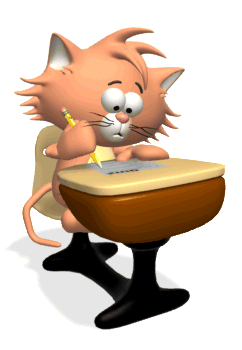• Testing Procedures1.  Tests are given at the end of a unit after all of the required lessons have been covered thoroughly.  If we are strictly using the Coach book, tests will be given after the end of a category.  2.  Quizzes are given randomly.  Students usually know about the quiz 1-2 days in advance, however "pop" quizzes may be given.     3.  Times Table quizzes will be given periodically throughout the year.  Students will be expected to write the problems they get incorrect 25x each.  The 25x each will be used as a homework grade.